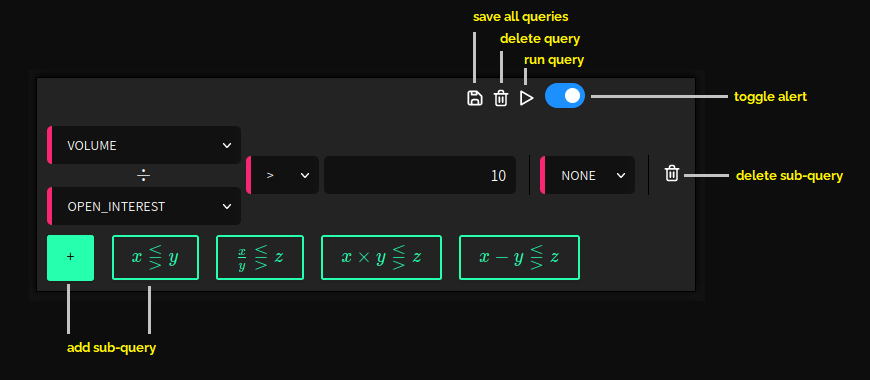## DocumentationEach query can have up to 20 sub-queries. In the figure above there is just one sub-query (a division query, since the two fields volume and open interest are divided), but more can be added by clicking the plus button and then selecting one of the four sub-query types. For now, there are four types: A simple comparison with a threshold (e.g. $volume > 100$), a division query (e.g. $\frac{volume}{open\_interest} > 10$), i.e. the two selected KPIs are divided, a multiplication query ($volume \times close > 10$), and a subtraction query (good for spreads: $ask - bid$). Sub-queries can be deleted again.